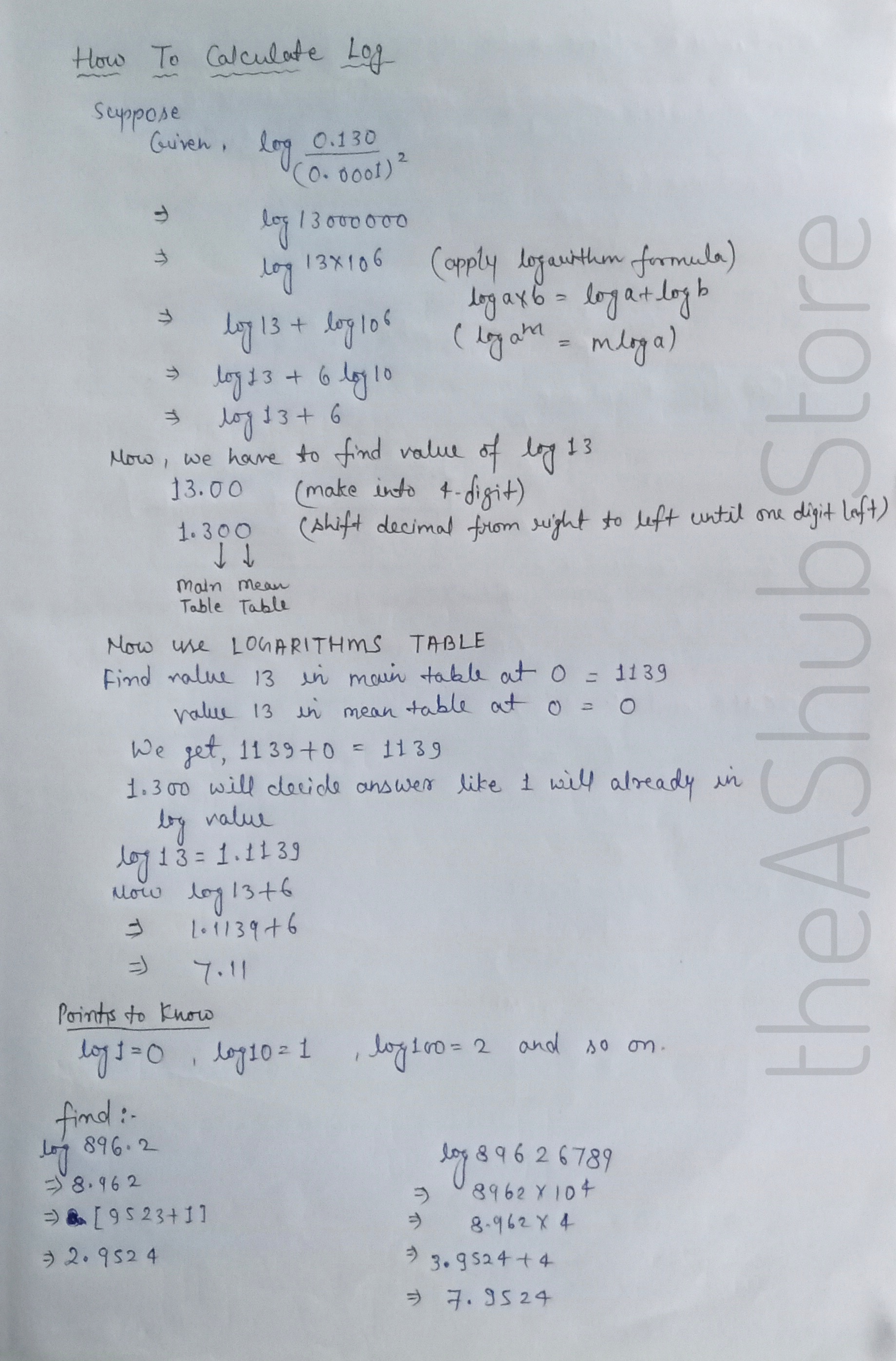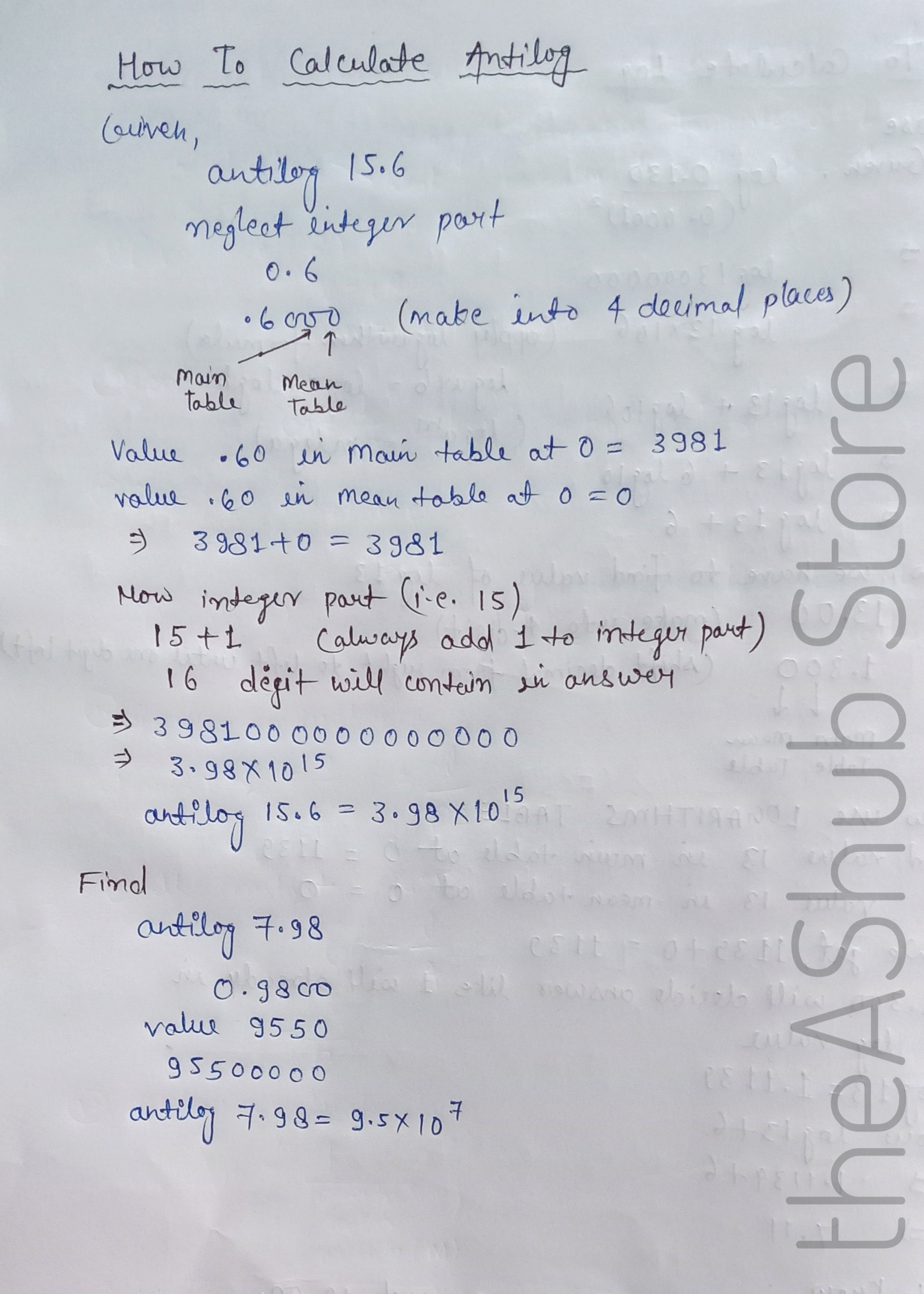# Calculate Log and Antilog within secondsIn this blog post I will show you how to calculate Log and Antilog so easily within seconds as it consists an important section in calculation of mathematics, chemistry, physics.
Logarithms, a way of writing the exponents. A logarithm of a number with a base is equal to another number. It is just the opposite function of exponentiation.
For example,
if 102 = 100,  then log10 100 = 2

Basically logarithm are two different types,
Common Logarithm
Natural Logarithm
Common Logarithm
It is also called the base 10 logarithms. It is represented as log10 or simply log.
Natural Logarithm
The natural logarithm is called the base e logarithm. The natural logarithm is represented as ln or loge.
Here, “e” represents the Euler’s constant which is approximately equal to 2.71828.
Before calculation of logarithm and anti-logarithm we should know about the parts like the characteristic and mantissa part.
Characteristic Part – The whole part is called the characteristic part.
Mantissa Part – The decimal part of the logarithm number for a given number is called the mantissa part.
For example. 10.5
Characteristics (integer) part =10
Mantissa = 5
How to calculate Log ?Antilog- The Antilog, also “Anti- Logarithms” of a number is the inverse technique of finding the logarithm of the same number.
How to calculate Antilog ?